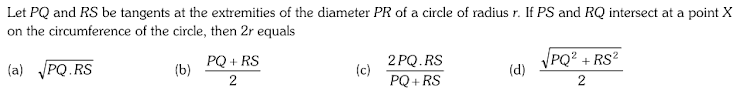CHECK POINTS ON CIRCLE
There is no negative marking. 4 marks will be given for every correct answer. Good luck.
NAME *
MOBILE NUMBER *
Q.1 Standard forms of Equation of a Circle.(1) General equation of a circle : (2) Central form of equation of a circle : (3) Concentric circle : (4) Circle on a given diameter : (5) Parametric coordinates (6) Equation of a circle under given conditions: Cyclic quadrilateral : Equation of a Circle in Some special cases.Circumcircle of a triangle
4 points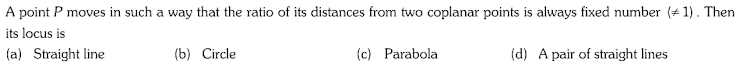Q.2 Standard forms of Equation of a Circle.(1) General equation of a circle : (2) Central form of equation of a circle : (3) Concentric circle : (4) Circle on a given diameter : (5) Parametric coordinates (6) Equation of a circle under given conditions: Cyclic quadrilateral : Equation of a Circle in Some special cases.Circumcircle of a triangle
4 points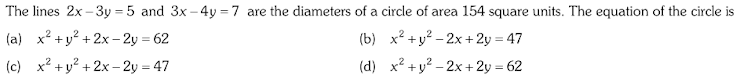Q.3 Standard forms of Equation of a Circle.(1) General equation of a circle : (2) Central form of equation of a circle : (3) Concentric circle : (4) Circle on a given diameter : (5) Parametric coordinates (6) Equation of a circle under given conditions: Cyclic quadrilateral : Equation of a Circle in Some special cases.Circumcircle of a triangle
4 points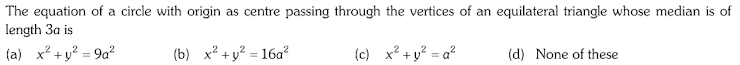Q.4 Standard forms of Equation of a Circle.(1) General equation of a circle : (2) Central form of equation of a circle : (3) Concentric circle : (4) Circle on a given diameter : (5) Parametric coordinates (6) Equation of a circle under given conditions: Cyclic quadrilateral : Equation of a Circle in Some special cases.Circumcircle of a triangle
4 points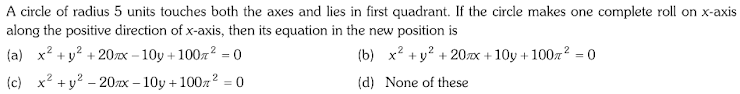Q.5 Standard forms of Equation of a Circle.(1) General equation of a circle : (2) Central form of equation of a circle : (3) Concentric circle : (4) Circle on a given diameter : (5) Parametric coordinates (6) Equation of a circle under given conditions: Cyclic quadrilateral : Equation of a Circle in Some special cases.Circumcircle of a triangle
4 points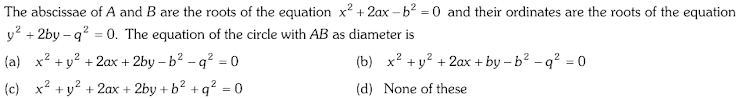Q.6 Standard forms of Equation of a Circle.(1) General equation of a circle : (2) Central form of equation of a circle : (3) Concentric circle : (4) Circle on a given diameter : (5) Parametric coordinates (6) Equation of a circle under given conditions: Cyclic quadrilateral : Equation of a Circle in Some special cases.Circumcircle of a triangle
4 points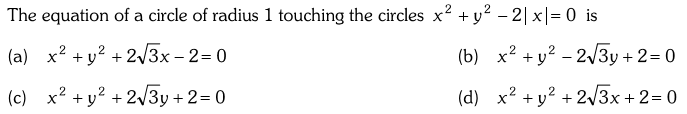Q.7 Standard forms of Equation of a Circle.(1) General equation of a circle : (2) Central form of equation of a circle : (3) Concentric circle : (4) Circle on a given diameter : (5) Parametric coordinates (6) Equation of a circle under given conditions: Cyclic quadrilateral : Equation of a Circle in Some special cases.Circumcircle of a triangle
4 points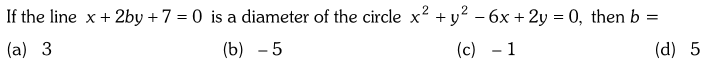Q.8 Standard forms of Equation of a Circle.(1) General equation of a circle : (2) Central form of equation of a circle : (3) Concentric circle : (4) Circle on a given diameter : (5) Parametric coordinates (6) Equation of a circle under given conditions: Cyclic quadrilateral : Equation of a Circle in Some special cases.Circumcircle of a triangle
4 points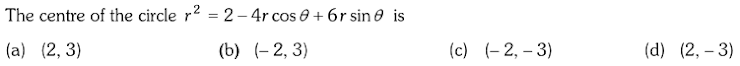Q.9 Standard forms of Equation of a Circle.(1) General equation of a circle : (2) Central form of equation of a circle : (3) Concentric circle : (4) Circle on a given diameter : (5) Parametric coordinates (6) Equation of a circle under given conditions: Cyclic quadrilateral : Equation of a Circle in Some special cases.Circumcircle of a triangle
4 points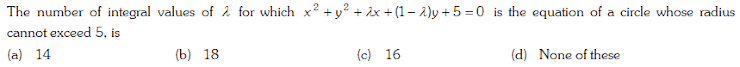Q.10 Standard forms of Equation of a Circle.(1) General equation of a circle : (2) Central form of equation of a circle : (3) Concentric circle : (4) Circle on a given diameter : (5) Parametric coordinates (6) Equation of a circle under given conditions: Cyclic quadrilateral : Equation of a Circle in Some special cases.Circumcircle of a triangle
4 points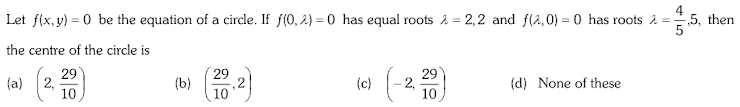Q.11 Standard forms of Equation of a Circle.(1) General equation of a circle : (2) Central form of equation of a circle : (3) Concentric circle : (4) Circle on a given diameter : (5) Parametric coordinates (6) Equation of a circle under given conditions: Cyclic quadrilateral : Equation of a Circle in Some special cases.Circumcircle of a triangle
4 pointsQ.12 Intercepts on the Axes. 4.5 Position of a point with respect to a Circle.(1) The least and greatest distance of a point from a circle :
4 points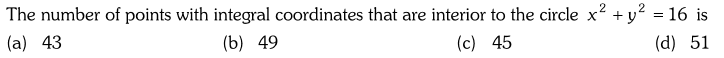Q.13 Intercepts on the Axes. 4.5 Position of a point with respect to a Circle.(1) The least and greatest distance of a point from a circle :
4 points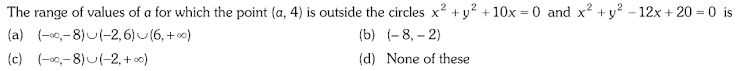Q.14 Intersection of a Line and a Circle.
4 points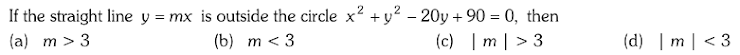Q.15 Intersection of a Line and a Circle.
4 points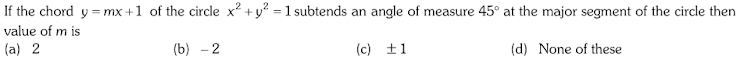Q.16 Tangent to a Circle at a given Point.(1) Point form (2) Parametric form : (3) Slope form : (4) Point of contact :
4 points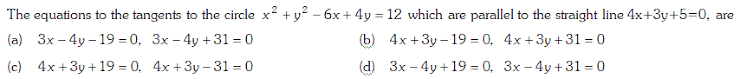Q.17 Tangent to a Circle at a given Point.(1) Point form (2) Parametric form : (3) Slope form : (4) Point of contact :
4 points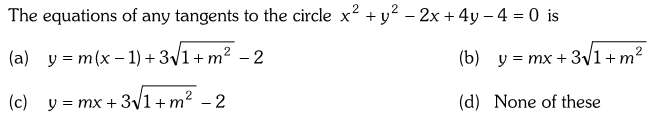Q.18 Tangent to a Circle at a given Point.(1) Point form (2) Parametric form : (3) Slope form : (4) Point of contact :
4 pointsQ.19 Length of Tangent, Pair of Tangents, Power of Point with respect to a Circle.
4 points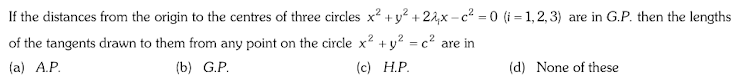Q.20 Length of Tangent, Pair of Tangents, Power of Point with respect to a Circle.
4 points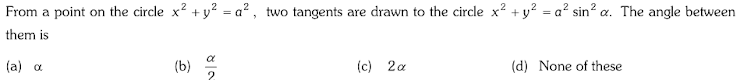Q.21 Length of Tangent, Pair of Tangents, Power of Point with respect to a Circle.
4 points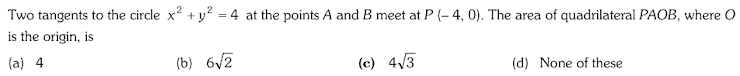Q.22 Length of Tangent, Pair of Tangents, Power of Point with respect to a Circle.
4 points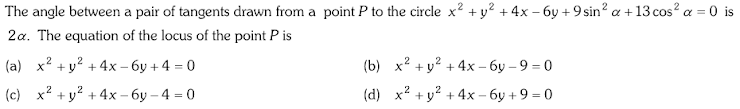Q.23 Normal to a Circle at a given Point.(1) Equation of normal: (2) Parametric form :
4 pointsQ.24 Chord of Contact of Tangents
4 points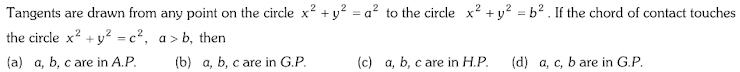Q.25 Chord of Contact of Tangents
4 points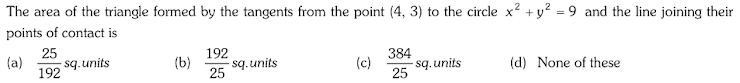Q.26 Chord of Contact of Tangents
4 points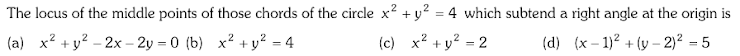Q.27 Chord of Contact of Tangents
4 points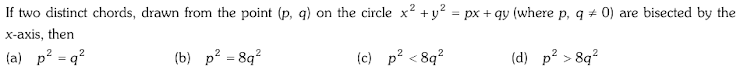Q.28 Director Circle, Diameter of a Circle
4 points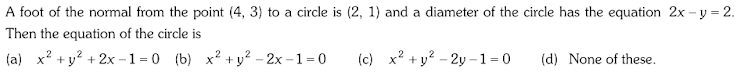Q.29 Director Circle. 4.14 Diameter of a Circle
4 points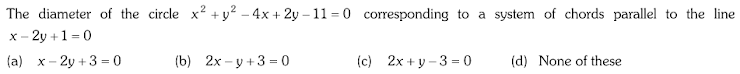Q.30 Pole and Polar.(1) Coordinates of pole of a line : (2) Properties of pole and polar
4 points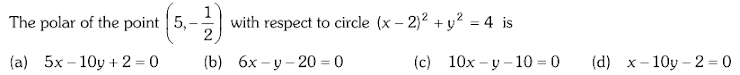Q.31 Pole and Polar.(1) Coordinates of pole of a line : (2) Properties of pole and polar
4 points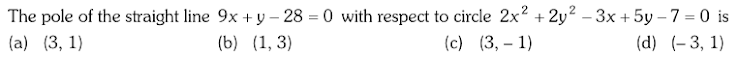Q.32 Two Circles touching each other, When two circles touch each other externally: When two circles touch each other internally : Common Tangents to Two circles.
4 points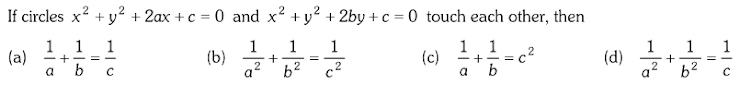Q.33 Two Circles touching each other, When two circles touch each other externally: When two circles touch each other internally : Common Tangents to Two circles.
4 points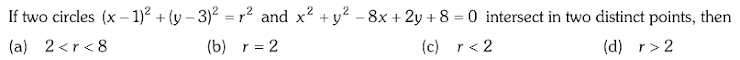Q.34 Two Circles touching each other, When two circles touch each other externally: When two circles touch each other internally : Common Tangents to Two circles.
4 points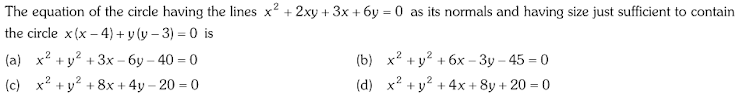Q.35 Two Circles touching each other, When two circles touch each other externally: When two circles touch each other internally : Common Tangents to Two circles.
4 points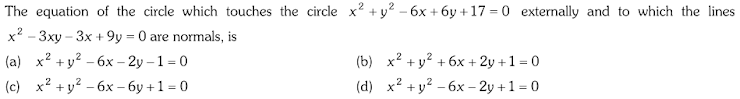Q.36 Two Circles touching each other, When two circles touch each other externally: When two circles touch each other internally : Common Tangents to Two circles.
4 points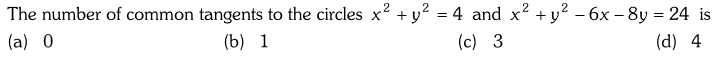Q.37 Two Circles touching each other, When two circles touch each other externally: When two circles touch each other internally : Common Tangents to Two circles.
4 points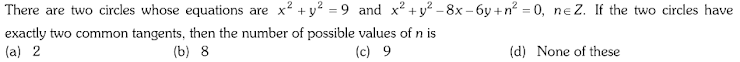Q.38 Common chord of two Circles.
4 pointsQ.39 Common chord of two Circles.
4 points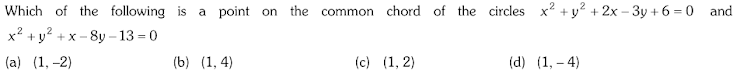Q.40 Common chord of two Circles.
4 points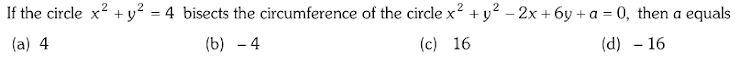Q.41 Angle of Intersection of Two Circles, Condition of Orthogonality :
4 points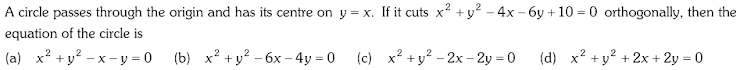Q.42 Angle of Intersection of Two Circles, Condition of Orthogonality :
4 points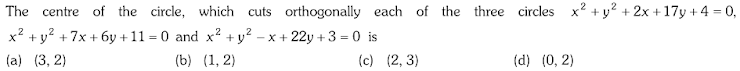Q.43 Angle of Intersection of Two Circles, Condition of Orthogonality :
4 points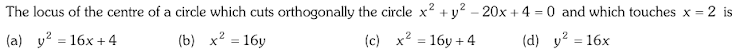Q.44 Family of Circles
4 points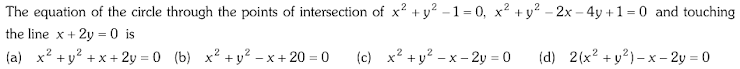Q.45 Family of Circles
4 points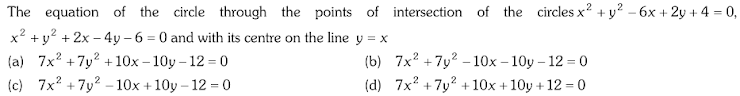4 points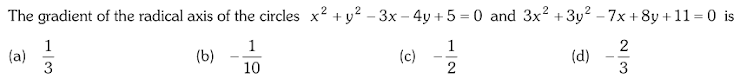4 points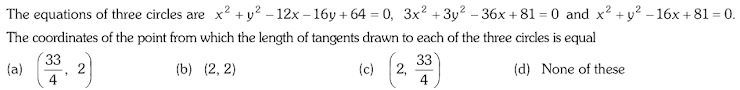4 points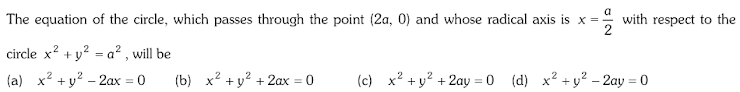Q.49 Co-Axial System of Circles, Limiting Points, Limiting points of the co-axial system : System of co-axial circles whose two limiting points are given : Properties of limiting points
4 points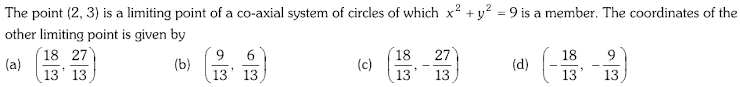Q.50 Co-Axial System of Circles, Limiting Points, Limiting points of the co-axial system : System of co-axial circles whose two limiting points are given : Properties of limiting points
4 points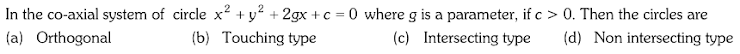Q.51 Co-Axial System of Circles, Limiting Points, Limiting points of the co-axial system : System of co-axial circles whose two limiting points are given : Properties of limiting points
4 pointsQ.52 Some Important Results, Concyclic points :
4 points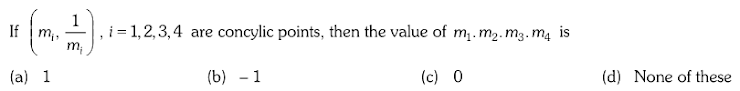Q.53 Some Important Results, Concyclic points :
4 points